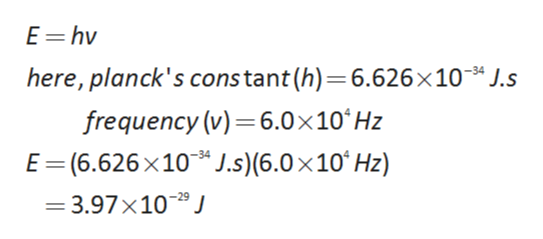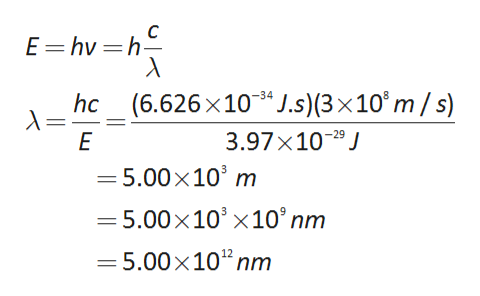# What is the wavelength, in nanometers, of an electromagnetic wave that has a frequency of 6.0 × 10^4 HZ? (1nm=10^-9m)

Question
1 views

What is the wavelength, in nanometers, of an electromagnetic wave that has a frequency of 6.0 × 10^4 HZ? (1nm=10^-9m)

check_circle

star
star
star
star
star
1 Rating
Step 1

The calculation of energy  as follows:help_outlineImage TranscriptioncloseE=hv here, planck's constant (h)=6.626×10* J.s frequency (v)=6.0×10ʻHZ E=(6.626x10*J.s)(6.0×10ʻHz) -34 %3D -34 = 3.97×10-®J fullscreen
Step 2

Then wavelength can be...help_outlineImage TranscriptioncloseE= hv =h- %3D hc_ (6.626x10* J.s)(3×10°m / s) 3.97×10-º J -34 = 5.00x10' m = 5.00x10' ×10° nm = 5.00x10"nm fullscreen

### Want to see the full answer?

See Solution

#### Want to see this answer and more?

Solutions are written by subject experts who are available 24/7. Questions are typically answered within 1 hour.*

See Solution
*Response times may vary by subject and question.
Tagged in